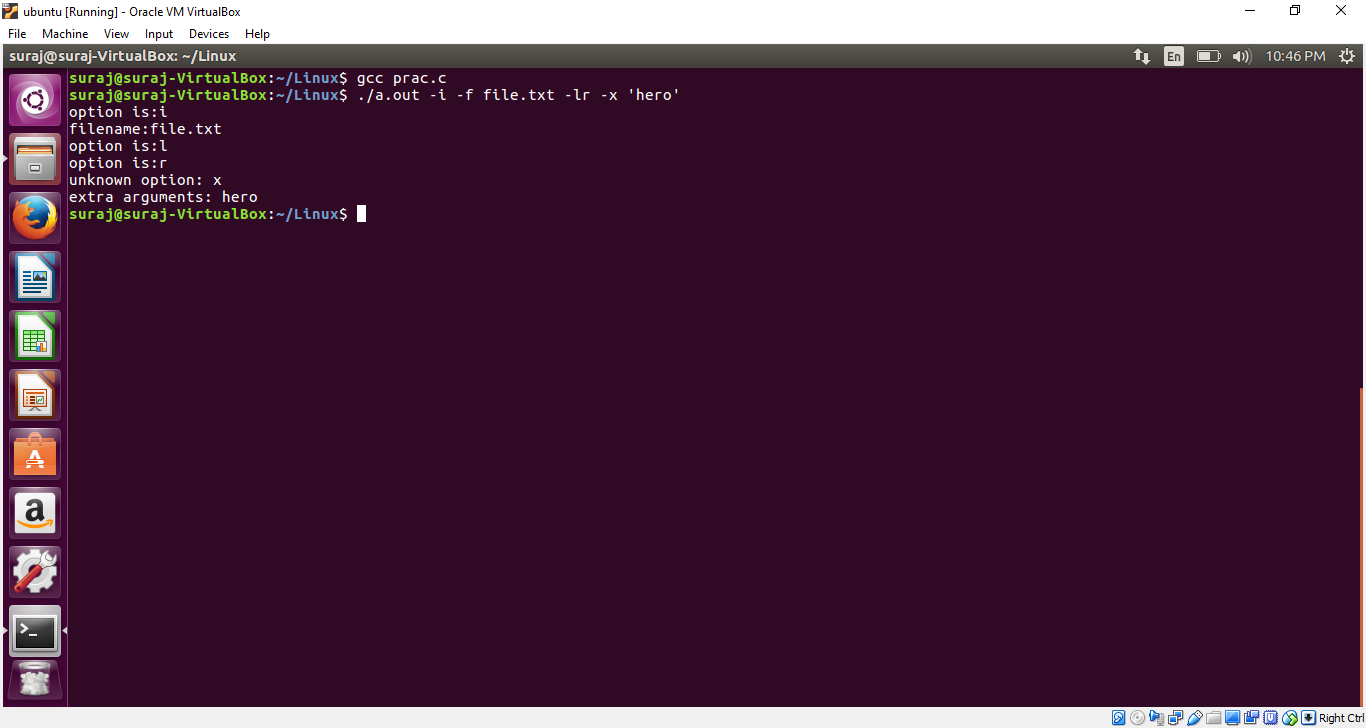Open in App
Not now

# getopt() function in C to parse command line arguments

• Difficulty Level : Easy
• Last Updated : 10 Sep, 2018

The getopt() function is a builtin function in C and is used to parse command line arguments.

Syntax:

```getopt(int argc, char *const argv[], const char *optstring)

optstring is simply  a list of characters,
each representing a single character option.
```

Return Value: The getopt() function returns different values:

• If the option takes a value, that value is pointer to the external variable optarg.
• ‘-1’ if there are no more options to process.
• ‘?’ when there is an unrecognized option and it stores into external variable optopt.
• If an option requires a value (such as -f in our example) and no value is given, getopt normally returns ?.
By placing a colon as the first character of the options string, getopt returns: instead of ? when no value is given.

Generally, the getopt() function is called from inside of a loop’s conditional statement. The loop terminates when the getopt() function returns -1. A switch statement is then executed with the value returned by getopt() function.

A second loop is used to process the remaining extra arguments that cannot be processed in the first loop.

Below program illustrate the getopt() function in C:

 `// Program to illustrate the getopt()``// function in C`` ` `#include  ``#include  `` ` `int` `main(``int` `argc, ``char` `*argv[]) ``{``    ``int` `opt;``     ` `    ``// put ':' in the starting of the``    ``// string so that program can ``    ``//distinguish between '?' and ':' ``    ``while``((opt = getopt(argc, argv, “:``if``:lrx”)) != -1) ``    ``{ ``        ``switch``(opt) ``        ``{ ``            ``case` `‘i’: ``            ``case` `‘l’: ``            ``case` `‘r’: ``                ``printf``(“option: %c\n”, opt); ``                ``break``; ``            ``case` `‘f’: ``                ``printf``(“filename: %s\n”, optarg); ``                ``break``; ``            ``case` `‘:’: ``                ``printf``(“option needs a value\n”); ``                ``break``; ``            ``case` `‘?’: ``                ``printf``(“unknown option: %c\n”, optopt);``                ``break``; ``        ``} ``    ``} ``     ` `    ``// optind is for the extra arguments``    ``// which are not parsed``    ``for``(; optind < argc; optind++){     ``        ``printf``(“extra arguments: %s\n”, argv[optind]); ``    ``}``     ` `    ``return` `0;``}`

Output:My Personal Notes arrow_drop_up